The CKN Knowledge in Practice Centre is in the early stages of content creation and currently focuses on the theme of thermal management.
We appreciate any feedback or content suggestions/requests using the links below

# Micro-Mechanics - A245

Micro-Mechanics
Foundational knowledge articleDocument Type Article
Document Identifier 245
Tags
Prerequisites

## Overview

The mechanics of composite materials is inherently complex as it involves the interaction between multiple different constituent materials. Prediction of a composite material’s mechanical behaviour involves analysis at several size scales: micro-, mes-, and macro- mechanics.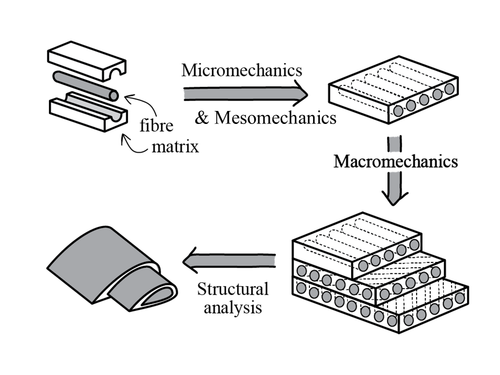The mechanical analysis of composite materials spans multiple size-scales. Analysis involves scales ranging from the small micro- and mesoscale, up to the larger macro-, and structural scale.

Micro and mesomechanics allows for the determination of elastic moduli at the ply or lamina level for a composite material.

## Coordinate System Conventions

The coordinate system conventions for each lamina is dictated by the orientation of fibers, independent of the x, y & z of the combined laminate. The convention is to use 1, 2 & 3 as directions, where 1 is the along the direction of fibers, 2 is perpendicular to the fibers and 3 is out of plane perpendicular to the fibers. This is to allow for accurate description of each lamina and predict strains as the lamina is loaded in different directions. See figure for illustration of fibers and coordinate conventions.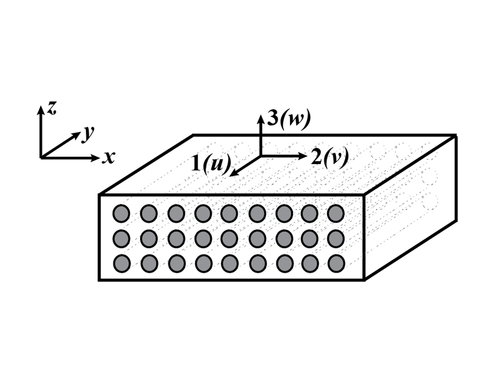At the micromechanics level, the 1-direction is conventionally used to refer to the fibre axial direction. The 2-direction is conventionally used to refer to the transverse fibre direction, in-plane in the lamina. While the 3-direction is conventionally used to refer to the transverse fibre, out-of-plane direction.

1 - fibre direction

2 - transverse fibre direction, in-plane

3 - out-of-plane

u, v, w – displacements in 1, 2, 3 directions respectively

## Plane Stress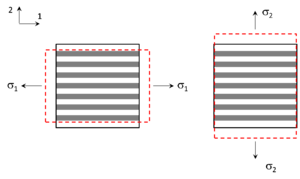Illustration of Poisson's ratio for an orthotropic laminae being loaded in the one and the 2 direction.

Plane stress is when a body is loaded in one axis only and is thusly only subject to two stresses. This is an assumption which is true only if the thickness of the body is small. If the material is isotropic, the result is that the body will be stretched in the direction of the force and the two remaining sides will contract inwards, see figure. This is the basic property that builds to Poisson’s ratio.

Plane stresses in orthotropic materials such as fiber reinforced lamina have a different perpendicular strain depending on which direction it is loaded in. This is due to the fact that the fibre and matrix have different properties and so are able to resist or promote strain depending on the direction of loading. When a fibre lamina is loaded in the 1 direction, the fibres are being pulled, allowing the matrix to experience the compressive strain. However, when the lamina is loaded in the 2 direction the fibres are able to resist the perpendicular strain. This leads orthotropic materials to have more than one Poisson’s ratio that is dependent on direction. The two ratios are often referred to as major and minor Poisson’s ratio, where major refers to loading in the 1 direction and larger strains $$(\nu_{12})$$ and minor is loading in the 2 direction where the compressive strain is lower $$(\nu_{21})$$

## Shear Coupling

It is through a continuation of this that we see when the lamina is loaded at an angle to the fibers, the different properties of the fibers and matrix cause coupled deformation. This means that when the fibers are at an angle to the stress, they withstand strain along their direction, but the matrix will deform perpendicularly to the fibers. This causes and extension and shear deformation to happen simultaneously also known as shear coupling, see figure for example.

## Engineering Constants

At the lamina level (a single laminate ply) or if all the reinforcement material is continuous and running in a single direction, a rule of mixtures approach can be applied when doing analysis of the various elastic moduli. In this approach, an approximation of the composite properties is obtained by using volume-weighted averages of the various composite constituents.

### Tensile Young's Modulus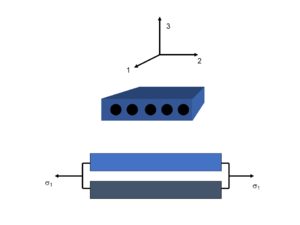Micromechanics 1-direction analysis (fibre direction) for continuous and unidirectional fibre composites. Equal stain is observed for the fibre and matrix.

Continuous unidirectional fibre reinforcement, fibre direction (1-direction):

$$E_1={E_f}{V_f}+{E_m}{V_m}$$

Where,

$$E_f =$$ Fibre tensile Young's modulus

$$V_f =$$ Fibre volume fraction

$$E_m =$$ Matrix tensile Young's modulus

$$V_m =$$ Matrix volume fraction

Continuous unidirectional fibre reinforcement, transverse fibre direction (2-direction):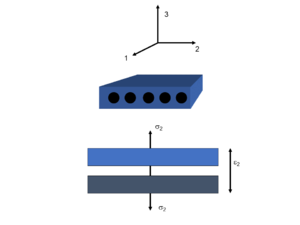Micromechanics 2-direction analysis (transverse fibre direction) for continuous and unidirectional fibre composites. Equal stress is observed for the fibre and matrix.

$$\frac{1}{E_2}=\frac{1}{E_f}{V_f}+\frac{1}{E_m}{V_m}$$

Where,

$$E_f =$$ Fibre tensile Young's modulus

$$V_f =$$ Fibre volume fraction

$$E_m =$$ Matrix tensile Young's modulus

$$V_m =$$ Matrix volume fraction

### Shear Modulus

Continuous unidirectional fibre reinforcement, in-plane (1,2-direction):

$$\frac{1}{G_{12}}=\frac{1}{G_f}{V_f}+\frac{1}{G_m}{V_m}$$

Where,

$$G_f =$$ Fibre shear modulus

$$V_f =$$ Fibre volume fraction

$$G_m =$$ Matrix shear modulus

$$V_m =$$ Matrix volume fraction

### Engineering Constants as a Function of Angle

For an isotropic material, engineering constants such as Young’s modulus (E) and shear modulus (G) are considered the same in all directions. However, in a lamina the Young’s modulus, for example, is much higher in the direction of the fibers than in the 2 direction. This leads to that the engineering constants in a lamina are a function of fiber orientation in relation to the direction of stress applied. The E modulus is high in fiber direction and the G modulus is highest at 45 degrees to the fibers, as well as the Poisson’s ratio changing with the angle.

## Hooke's Law

The first step to understanding mechanics in composites is to understand Hooke's Law for an isotropic material. Hooke’s law is used to define plane stress in an isotropic material undergoing uniaxial load, it describes the relationship between stress and strain in linear elasticity. Hooke’s Law states that stress and strain are linearly proportional for small strains. For an isotropic material Hooke’s law can be expressed as: $\varepsilon_{1}=\frac{\sigma_{1}}{E}$ Recalling that Poisson’s ratio is defined as: $v=-\frac{\varepsilon_{2}}{\varepsilon_{1}}$ Hooke’s Law can be written in this form to predict strain in the 2 direction: $\varepsilon_{2}=-v \varepsilon_{1}=-v \frac{\sigma_{1}}{E}$ The effect of this is illustrated by the figure to the right.

When stresses are present in the 1 and the 2 direction in a biaxial loading situation, the strains can be expressed as: \begin{aligned} &\varepsilon_{1}=\frac{\sigma_{1}}{E}-v \frac{\sigma_{2}}{E} \\ &\varepsilon_{2}=-v \frac{\sigma_{1}}{E}+\frac{\sigma_{2}}{E} \end{aligned}

Hooke’s law can also be used for shear loading by using the shear modulus, G, instead of young’s modulus: $G=\frac{E}{2(1+v)}$ The shear strain, $$\gamma_{12}$$ can then be defined in terms of shear modulus, $$G$$, and shear load, $$\tau_{12}$$ as: $\gamma_{12}=\frac{\tau_{12}}{G}$

All of the previous expressions can be combined to form a compliance [S] and stiffness [Q] matrix to account for all loads and effects it can have on the isotropic material. Where the stiffness matrix is the inverse of the compliance matrix. Hooke’s law can then be presented as $$\{\varepsilon\}=[s]\{\sigma\}$$ and $$\{\sigma\}=[Q]\{\varepsilon\}$$.

The expanded form of the compliance matrix is then: $\left\{\begin{array}{l} \varepsilon_{1} \\ \varepsilon_{2} \\ \gamma_{12} \end{array}\right\}=\left[\begin{array}{ccc} \frac{1}{E} & \frac{-v}{E} & 0 \\ \frac{-v}{E} & \frac{1}{E} & 0 \\ 0 & 0 & \frac{1}{G} \end{array}\right]\left\{\begin{array}{l} \sigma_{1} \\ \sigma_{2} \\ \tau_{12} \end{array}\right\}$

and the expanded stiffness matrix becomes: $\left\{\begin{array}{l} \sigma_{1} \\ \sigma_{2} \\ \tau_{12} \end{array}\right\}=\left[\begin{array}{ccc} \frac{E}{1-v^{2}} & \frac{v E}{1-v^{2}} & 0 \\ \frac{v E}{1-v^{2}} & \frac{E}{1-v^{2}} & 0 \\ 0 & 0 & G \end{array}\right]\left\{\begin{array}{l} \varepsilon_{1} \\ \varepsilon_{2} \\ \gamma_{12} \end{array}\right\}$

### Orthotropic Material

For an orthotropic composite material, the strain experienced is dependent on the direction of loading due to the fibers either resisting the strain or not being involved. Hooke’s law and the underlying principles can still be applied, but the stiffness and Poisson’s ratio are different for the 1 and 2 directions. This leads to the strain equations being: $\varepsilon_{2}=-v_{12} \varepsilon_{1}=-v_{12} \frac{\sigma_{1}}{E_{1}}$ $\varepsilon_{1}=-v_{21} \varepsilon_{2}=-v_{21} \frac{\sigma_{2}}{E_{2}}$ Giving the compliance matrix, [S], to be: $\left\{\begin{array}{l} \varepsilon_{1} \\ \varepsilon_{2} \\ \gamma_{12} \end{array}\right\}=\left[\begin{array}{ccc} \frac{1}{E_{1}} & \frac{-v_{12}}{E_{1}} & 0 \\ \frac{-v_{12}}{E_{1}} & \frac{1}{E_{2}} & 0 \\ 0 & 0 & \frac{1}{G_{12}} \end{array}\right]\left\{\begin{array}{l} \sigma_{1} \\ \sigma_{2} \\ \tau_{12} \end{array}\right\}$

and the stiffness matrix, [Q], is: $\left\{\begin{array}{l} \sigma_{1} \\ \sigma_{2} \\ \tau_{12} \end{array}\right\}=\left[\begin{array}{ccc} \frac{E_{1}}{1-v_{21}v_{12}} & \frac{v_{12} E_{2}}{1-v_{21}v_{12}} & 0 \\ \frac{v_{12} E_{2}}{1-v_{21}v_{12}} & \frac{E_{2}}{1-v_{21}v_{12}} & 0 \\ 0 & 0 & G_{12} \end{array}\right]\left\{\begin{array}{l} \varepsilon_{1} \\ \varepsilon_{2} \\ \gamma_{12} \end{array}\right\}$

## Angle Lamina

### Transformation Matrix and Inverse Transformation Matrix

There will be situations where the lamina 1,2 and 3 directions do not align with the loading axes x, y, and z. In order to compensate for this shift and continue to calculate the properties of a lamina, the elastic properties need to be transformed from the local coordinate system to the global one. A transformation matrix, [T], is established in terms of $$\sin\theta$$ and $$\cos\theta$$ to rotate the x and y coordinate system counter clockwise to align with the local 1 and 2 coordinate system. Defining $$s=\sin\theta$$ and $$c=\cos\theta$$ the transformation matrix for stresses becomes: $\left\{\begin{array}{l} \sigma_{1} \\ \sigma_{2} \\ \tau_{12} \end{array}\right\}=[T]\left\{\begin{array}{l} \sigma_{x} \\ \sigma_{y} \\ \tau_{x y} \end{array}\right\}\rightarrow [T]=\left[\begin{array}{ccc} c^{2} & s^{2} & 2 s c \\ s^{2} & c^{2} & -2 s c \\ -s c & s c & c^{2}-s^{2} \end{array}\right]$

The strain transformation matrix [T*] is similarly: $\left\{\begin{array}{c} \varepsilon_{1} \\ \varepsilon_{2} \\ \gamma_{12} \end{array}\right\}=\left[T^{*}\right]\left\{\begin{array}{c} \varepsilon_{x} \\ \varepsilon_{y} \\ \gamma_{x y} \end{array}\right\}\rightarrow\left[T^{*}\right]=\left[\begin{array}{ccc} c^{2} & s^{2} & s c \\ s^{2} & c^{2} & -s c \\ -2 s c & 2 s c & c^{2}-s^{2} \end{array}\right]$

To rotate the 1 and 2 coordinate system clockwise instead of the x and y, the same principles can be applied by using the inverse of the transformation matrix: $\left\{\begin{array}{l} \sigma_{x} \\ \sigma_{y} \\ \tau_{x y} \end{array}\right\}=[T]^{-1}\left\{\begin{array}{l} \sigma_{1} \\ \sigma_{2} \\ \tau_{12} \end{array}\right\}\rightarrow[T]^{-1}=\left[\begin{array}{ccc} c^{2} & s^{2} & -2 s c \\ s^{2} & c^{2} & 2 s c \\ s c & -s c & c^{2}-s^{2} \end{array}\right]$

### Hooke's Law for Angle Lamina ($$\overline{S}$$ and $$\overline{Q}$$)

Since we have established Hooke’s law for lamina properties in the 1 and 2 direction and then developed a way to transform the stresses for an angled lamina to the different coordinate systems. It is now time to establish a method where stresses and strains in the x and y coordinate system can be directly calculated form an angled lamina. Meaning, a Hooke’s law relationship needs to be established with the transformation matrices included. The compliance matrix developed is referred to as $$\overline{[S]}$$: $\left.\left\{\begin{array}{c} \varepsilon_{x} \\ \varepsilon_{y} \\ \gamma_{x y} \end{array}\right\}=[\bar{S}] \begin{array}{c} \sigma_{x} \\ \sigma_{y} \\ \tau_{x y} \end{array}\right\}$

Starting from Hoke’s law and replacing the 1 and 2 coordinate system with transformed x and y systems give: $\left\{\begin{array}{l} \varepsilon_{1} \\ \varepsilon_{2} \\ \gamma_{12} \end{array}\right\}=[S]\left\{\begin{array}{l} \sigma_{1} \\ \sigma_{2} \\ \tau_{12} \end{array}\right\}\rightarrow\left[T^{*}\right]\left\{\begin{array}{l} \varepsilon_{x} \\ \varepsilon_{y} \\ \gamma_{x y} \end{array}\right\}=[S][T]\left\{\begin{array}{l} \sigma_{x} \\ \sigma_{y} \\ \tau_{x y} \end{array}\right\}$ Multiplying by the inverse of [T*] gives: $\left\{\begin{array}{l} \varepsilon_{x} \\ \varepsilon_{y} \\ \gamma_{x y} \end{array}\right\}=\left[T^{*}\right]^{-1}[S][T]\left\{\begin{array}{l} \sigma_{x} \\ \sigma_{y} \\ \tau_{x y} \end{array}\right\}$ Meaning that $$[\overline{S}] = [T^*]^{-1}[S][T]$$ The resulting matrix is then: $[\bar{S}]=\left[\begin{array}{ccc} \bar{S}_{11} & \bar{S}_{12} & \bar{S}_{16} \\ & \bar{S}_{22} & \bar{S}_{26} \\ S y m & & \bar{S}_{66} \end{array}\right]=\left[\begin{array}{ccc} \frac{1}{E_{x}} & -\frac{v_{x y}}{E_{x}} & -\frac{m_{x}}{E_{1}} \\ & \frac{1}{E_{y}} & -\frac{m_{y}}{E_{1}} \\ S y m & & \frac{1}{G_{x y}} \end{array}\right]$

Where [mx] and [my] are shear coupling parameters, see below. Note: "sym" in the matrix means that the matrix is mirrored diagonally, ie. $$\bar{S}_{12} = \bar{S}_{21}$$

Each engineering constant and the shear coupling parameters can then be established through the resulting matrix multiplication illustrated below: \begin{aligned} &\frac{1}{E_{x}}=\bar{S}_{11}=\frac{1}{E_{1}} c^{4}+\left(\frac{1}{G_{12}}-\frac{2 v_{12}}{E_{1}}\right) s^{2} c^{2}+\frac{1}{E_{2}} s^{4} \\ &\frac{1}{E_{y}}=\bar{S}_{22}=\frac{1}{E_{1}} s^{4}+\left(\frac{1}{G_{12}}-\frac{2 v_{12}}{E_{1}}\right) s^{2} c^{2}+\frac{1}{E_{2}} c^{4} \\ &\frac{1}{G_{x y}}=\bar{S}_{66}=2\left(\frac{2}{E_{1}}+\frac{2}{E_{2}}+\frac{4 v_{12}}{E_{1}}-\frac{1}{G_{12}}\right) s^{2} c^{2}+\frac{1}{G_{12}}\left(s^{4}+c^{4}\right) \\ &v_{x y}=-E_{x} \bar{S}_{12}=E_{x}\left[\frac{v_{12}}{E_{1}}\left(s^{4}+c^{4}\right)-\left(\frac{1}{E_{1}}+\frac{1}{E_{2}}-\frac{1}{G_{12}}\right) s^{2} c^{2}\right] \\ &m_{x}=-\bar{S}_{16} E_{1}=-E_{1}\left[\left(\frac{-2}{E_{1}}-\frac{-2 v_{12}}{E_{1}}+\frac{1}{G_{12}}\right) s c^{3}-\left(\frac{2}{E_{2}}+\frac{2 v_{12}}{E_{1}}-\frac{1}{G_{12}}\right) s^{3} c\right] \\ &m_{y}=-\bar{S}_{26} E_{1}=-E_{1}\left[\left(\frac{-2}{E_{1}}-\frac{-2 v_{12}}{E_{1}}+\frac{1}{G_{12}}\right) s^{3} c-\left(\frac{2}{E_{2}}+\frac{2 v_{12}}{E_{1}}-\frac{1}{G_{12}}\right) s c^{3}\right] \end{aligned}

The same concept can also be applied to the stiffness matrix: $\left\{\begin{array}{l} \sigma_{x} \\ \sigma_{y} \\ \tau_{x y} \end{array}\right\}=[\bar{Q}]\left\{\begin{array}{l} \varepsilon_{x} \\ \varepsilon_{y} \\ \gamma_{x y} \end{array}\right\}=\left[\begin{array}{lll} \bar{Q}_{11} & \bar{Q}_{12} & \bar{Q}_{16} \\ & \bar{Q}_{22} & \bar{Q}_{26} \\ S y m & & \bar{Q}_{66} \end{array}\right]\left\{\begin{array}{l} \varepsilon_{x} \\ \varepsilon_{y} \\ \gamma_{x y} \end{array}\right\}$

The shear coupling parameters are a result of a non-zero term in $$\bar{[S]}_{16}$$ and $$\bar{[S]}_{26}$$. This means that for a lamina that is being loaded at an angle to the fibers, there is a coupling between the shear and axial load direction, causing a distortion illustrated here:

### Engineering Constants as a Function of Angle

Since these matrices are a function of angle, the resulting engineering constants can be plotted to display how they change with angle:

### Engineering Constants for a Lamina

Below is a summary of this chapter in the form of a flowchart showing the process to find engineering constants for a lamina in x y coordinates of a load starting from the engineering constants in the 1 2 coordinates.

## Lamina Example Problem

This is the same example presented by Dr. Keulen in the 12 part webinar series on composite materials, which can be found here: Composite materials engineering webinar session 8 - Mechanics of composites - Part 1: Lamina level

A lamina with these properties and fibers at 60 degrees to the load direction. Find: Global strains, local strains, local stresses and global engineering constants.

Constant Value
Vf 0.70
E1 181 GPa
E2 10.30 GPa
v12 0.28
G12 7.17 GPa

1. Find the local compliance matrix $\left\{\begin{array}{l} \varepsilon_{1} \\ \varepsilon_{2} \\ \gamma_{12} \end{array}\right\}=\left[\begin{array}{ccc} \frac{1}{E} & \frac{-v}{E} & 0 \\ \frac{-v}{E} & \frac{1}{E} & 0 \\ 0 & 0 & \frac{1}{G} \end{array}\right]\left\{\begin{array}{l} \sigma_{1} \\ \sigma_{2} \\ \tau_{12} \end{array}\right\}$

\begin{aligned} &S_{11}=\frac{1}{181}=5.52 \cdot 10^{-3} \mathrm{GPa}^{-1} \\ &S_{12}=-\frac{0.28}{181}=-1.55 \cdot 10^{-3} \mathrm{GPa}^{-1} \\ &S_{22}=\frac{1}{10.3}=97.1 \cdot 10^{-3} \mathrm{GPa}^{-1} \\ &S_{66}=\frac{1}{7.17}=139 \cdot 10^{-3} \mathrm{GPa}^{-1} \end{aligned}\rightarrow[S]=\left[\begin{array}{ccc} 5.52 & -1.55 & 0 \\ & 97.1 & 0 \\ S y m & & 139 \end{array}\right] \cdot 10^{-3} \mathrm{GPa}^{-1}

2. Find the local stiffness matrix: $[Q]=[S]^{-1}=\left[\begin{array}{ccc} 181.8 & 2.897 & 0 \\ & 10.35 & 0 \\ S y m & & 7.17 \end{array}\right] \mathrm{GPa}$

3. Establish the transformation matrices: \begin{aligned} &{[T]=\left[\begin{array}{ccc} c^{2} & s^{2} & 2 s c \\ s^{2} & c^{2} & -2 s c \\ -s c & s c & c^{2}-s^{2} \end{array}\right]=\left[\begin{array}{ccc} 0.25 & 0.75 & 0.866 \\ 0.75 & 0.25 & -0.866 \\ -0.433 & 0.433 & -0.50 \end{array}\right]} \\\\ &{\left[T^{*}\right]=\left[\begin{array}{ccc} c^{2} & s^{2} & s c \\ s^{2} & c^{2} & -s c \\ -2 s c & 2 s c & c^{2}-s^{2} \end{array}\right]=\left[\begin{array}{ccc} 0.25 & 0.75 & 0.433 \\ 0.75 & 0.25 & -0.433 \\ -0.866 & 0.866 & -0.50 \end{array}\right]} \end{aligned}

4. The global compliance and stiffness matrices are then: $\begin{gathered} {[\bar{S}]=\left[T^{*}\right]^{-1}[S][T]=\left[\begin{array}{ccc} 80.53 & -7.878 & -32.34 \\ & 34.75 & -46.96 \\ S y m & & 114.1 \end{array}\right] \cdot 10^{-3} \mathrm{GPa}^{-1}} \\\\ {[\bar{Q}]=[\bar{S}]^{-1}=\left[\begin{array}{lll} 23.65 & 32.46 & 20.05 \\ & 109.4 & 54.19 \\ S y m & & 36.74 \end{array}\right] \mathrm{GPa}} \end{gathered}$

5. The global engineering constants are then found from the global compliance matrix: $\begin{array}{cl} \frac{1}{\bar{S}_{11}}=E_{x}=12.4 \mathrm{GPa} & v_{x y}=-E_{x} \bar{S}_{12}=0.0977 \\ \frac{1}{\bar{S}_{22}}=E_{y}=28.8 \mathrm{GPa} & m_{x}=-\bar{S}_{16} E_{1}=5.85 \\ \frac{1}{\bar{S}_{66}}=G_{x y}=8.76 \mathrm{GPa} & m_{y}=-\bar{S}_{26} E_{1}=8.50 \end{array}$

6. The global strains are calculated from the global compliance matrix as well: $\left\{\begin{array}{c} \varepsilon_{1} \\ \varepsilon_{2} \\ \gamma_{12} \end{array}\right\}=\left[\bar{S}\right]\left\{\begin{array}{c} 2\\ -3\\ 4 \end{array}\right\}MPa =\left\{\begin{array}{c} 55.34 \\ -307.8 \\ 532.8 \end{array}\right\} \mu \varepsilon$

7. The local strains are calculated using the strain transformation matrix: $\left\{\begin{array}{c} \varepsilon_{1} \\ \varepsilon_{2} \\ \gamma_{12} \end{array}\right\}=\left[T^{*}\right]\left\{\begin{array}{c} \varepsilon_{x} \\ \varepsilon_{y} \\ \gamma_{x y} \end{array}\right\}=\left\{\begin{array}{c} 13.67 \\ -266.2 \\ -580.9 \end{array}\right\} \mu \varepsilon$

8. Which can then be used to establish the local stresses, or they can be found by using the inverse transformation matrix and global stresses: $\left\{\begin{array}{c} \sigma_{1} \\ \sigma_{2} \\ \tau_{12} \end{array}\right\}=[Q\}\left\{\begin{array}{c} \varepsilon_{1} \\ \varepsilon_{2} \\ \gamma_{12} \end{array}\right\}=[T]^{-1}\left\{\begin{array}{c} \sigma_{x} \\ \sigma_{y} \\ \tau_{x y} \end{array}\right\}=\left\{\begin{array}{c} 1.714 \\ -2.714 \\ -4.165 \end{array}\right\} \mathrm{MPa}$

## Related pages

Introduction to Composites Articles
Foundational Knowledge Articles
Foundational Knowledge Method Documents
Foundational Knowledge Worked Examples
Systems Knowledge Articles
Systems Knowledge Method Documents
Systems Knowledge Worked Examples
Systems Catalogue Articles
Systems Catalogue Objects – Material
Systems Catalogue Objects – Shape
Systems Catalogue Objects – Tooling and consumables
Systems Catalogue Objects – Equipment
Practice Documents
Case Studies
Perspectives ArticlesAbout Help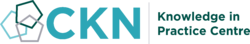## Welcome

Welcome to the CKN Knowledge in Practice Centre (KPC). The KPC is a resource for learning and applying scientific knowledge to the practice of composites manufacturing. As you navigate around the KPC, refer back to the information on this right-hand pane as a resource for understanding the intricacies of composites processing and why the KPC is laid out in the way that it is. The following video explains the KPC approach:

## Understanding Composites Processing

The Knowledge in Practice Centre (KPC) is centered around a structured method of thinking about composite material manufacturing. From the top down, the heirarchy consists of:

The way that the material, shape, tooling & consumables and equipment (abbreviated as MSTE) interact with each other during a process step is critical to the outcome of the manufacturing step, and ultimately critical to the quality of the finished part. The interactions between MSTE during a process step can be numerous and complex, but the Knowledge in Practice Centre aims to make you aware of these interactions, understand how one parameter affects another, and understand how to analyze the problem using a systems based approach. Using this approach, the factory can then be developed with a complete understanding and control of all interactions.

## Interrelationship of Function, Shape, Material & Process

Design for manufacturing is critical to ensuring the producibility of a part. Trouble arises when it is considered too late or not at all in the design process. Conversely, process design (controlling the interactions between shape, material, tooling & consumables and equipment to achieve a desired outcome) must always consider the shape and material of the part. Ashby has developed and popularized the approach linking design (function) to the choice of material and shape, which influence the process selected and vice versa, as shown below:

Within the Knowledge in Practice Centre the same methodology is applied but the process is more fully defined by also explicitly calling out the equipment and tooling & consumables. Note that in common usage, a process which consists of many steps can be arbitrarily defined by just one step, e.g. "spray-up". Though convenient, this can be misleading.

## Workflows

The KPC's Practice and Case Study volumes consist of three types of workflows:

• Development - Analyzing the interactions between MSTE in the process steps to make decisions on processing parameters and understanding how the process steps and factory cells fit within the factory.
• Troubleshooting - Guiding you to possible causes of processing issues affecting either cost, rate or quality and directing you to the most appropriate development workflow to improve the process
• Optimization - An expansion on the development workflows where a larger number of options are considered to achieve the best mixture of cost, rate & quality for your application.